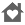# Mathstools Integral CalculatorEveryone
5
This app works with functions of one and two variables. Integral Calculator is designed for students and teachers in Maths, engineering, phisycs and sciences in general.

Application can resolve following maths operations:
- Symbolic primitive calculations.
- Integration.
- Symbolic derivate calculation.
- Plotting 2D and 3D functions.
- Zeros and inflection points calculation.
- Numerical integration approximations.
- Fourier Series numeric calculation.
- Limit Calculation.
- Symbolic equations solver x and y variables.

All of these calculations from mathstools.com libraries.
Internet access required!!.
Collapse

Review Policy
2.8
5 total
5
4
3
2
1

## What's New

System equations solver.
Matrix calculations.
Two-variabled functions plotter.
Equations solver.
Some more differential operators
Collapse

Eligible for Family LibraryEligible if bought after 7/2/2016. Learn More
Updated
December 10, 2015
Size
8.0M
Installs
100+
Current Version
1.65
Requires Android
2.3 and up
Content Rating
Everyone
Permissions
Offered By
Carlos Mathstools
Developer
calle jacinto gomez comesaña, 17 madrid 28038